# GIS Series Part 1/5: Spatial Reference Systems

Making sense of Spatial Reference Systems and their different identification numbers is probably one of the blurriest parts of dealing with GIS data. This is my attempt to provide a clear introduction, which I have tried to make as short as possible without losing too much information. In Part One of this series, we will explore the concept of a Spatial Reference System (SRS).

### 1- Projections

Flatteting our 3D earth into a plane to make a map has been causing smart people headaches for centuries.

In theory, a perfect map would allow you to measure quantities like areas, shapes, and distances accurately everywhere on earth. However, even if our planet was a perfect sphere (which it is really not), projecting a sphere on a plane will necessarily distort the surface in some way.

In the figure below, from Jochen Albrecht’s Geographic Information Science lecture notes, you can see how different projections distort different regions on earth.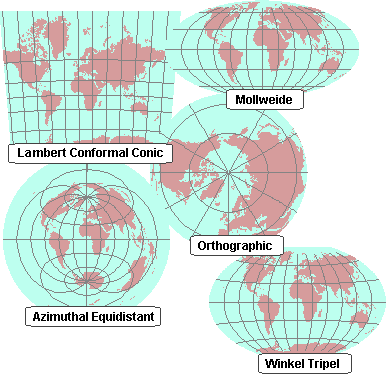Ultimately, you want to choose the projection that causes the least distortion for the region on earth that you care about.

### 2- The other half of the story

I started this post with projections because they are fairly intuitive. But let’s take a step back. Before we can project our 3D planet onto a surface, you must have a 3D model of the earth to begin with. Now remember when I told you that our planet is not a perfect sphere? Well, from the most recent approximations, its surface is a lot closer the potato-shaped object below called the geoid.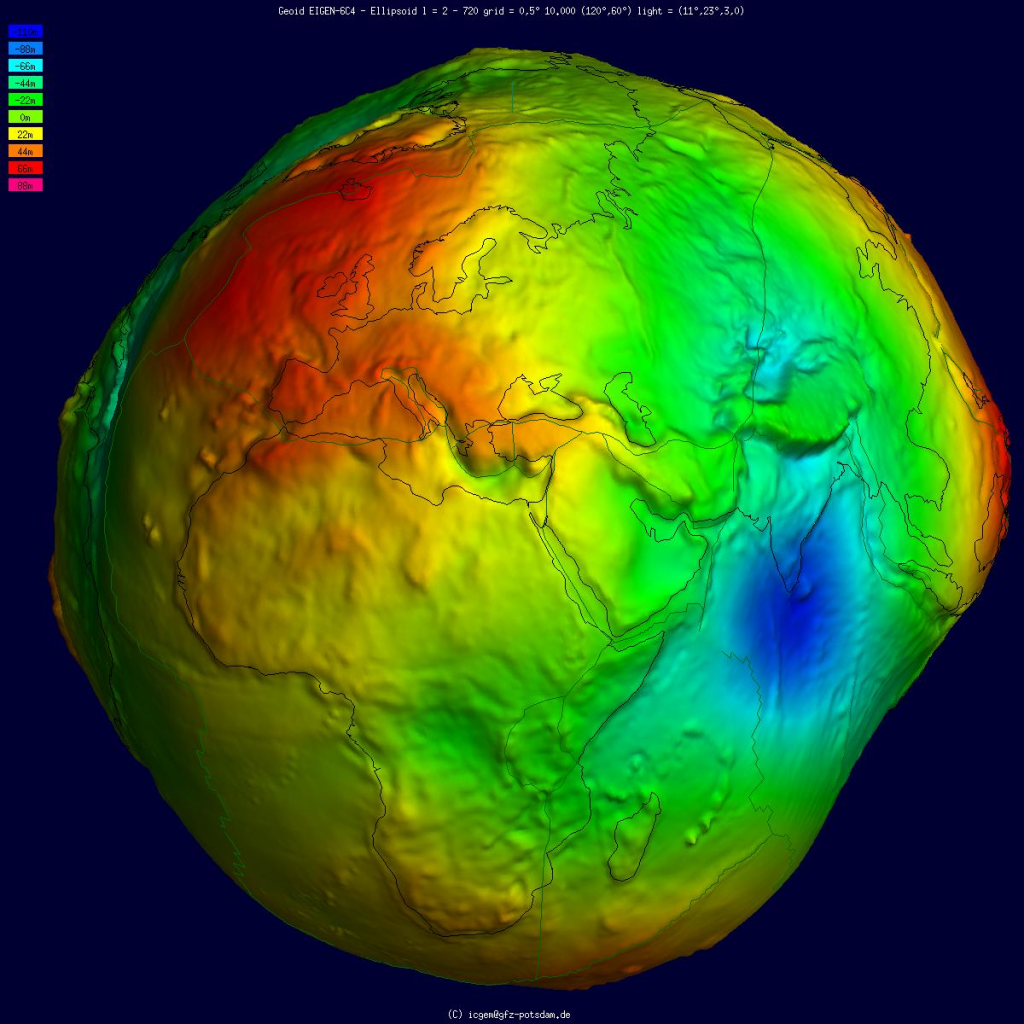So really what we want is to first, find the simplest 3D mathematical object that can approximate this potato, and then, project that mathematical object as accurately as possible for the region that you care about.

Let’s start with the simplest 2D mathematical object we can think of: a circle. The radius is the same along the x and y axis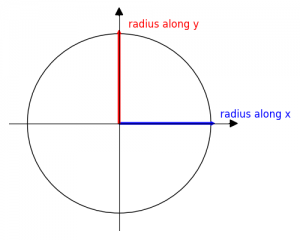To better approximate the uneven shape of the earth, we can make one side of the circle flatter than the other: you get an ellipse. The radius is no longer the same along the x and y axis.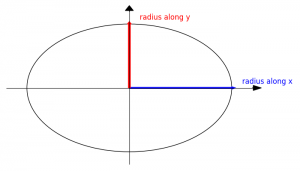Now find ellipse that best matches the curvature of the earth at the region that you want to map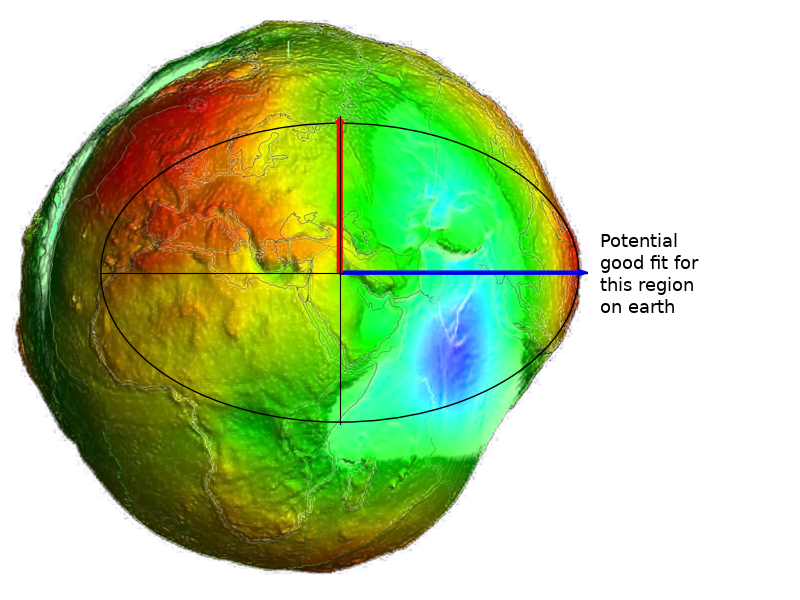Of course, to model our planet, you need to make the ellipse 3D: you get an ellipsoid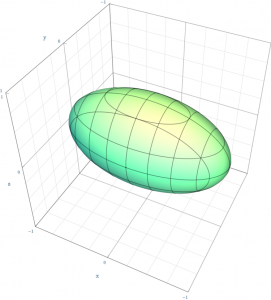A few more steps are needed to get a complete Spatial Reference System:

• pin the points where each axis of the ellipsoid reaches the surface of the earth: this is called the datum
• use a coordinate reference system to locate every point on the ellipsoid (the most popular coordinate reference system is longitude/latitude)

Now you can decide which projection you want to use to squash your ellipsoid into a 2D plane to work with. That’s it. You now have a fully defined Spatial Reference System.  🙂

### 3- In a Nutshell

Spatial Reference Systems (SRSs) are somewhat difficult to understand because they are composed of  several items:

• an ellipsoid that best matches the curvature of the earth for your region of interest
• a datum to pin the ellipsoid to actual points on the planet
• a coordinate system to locate every point on earth
• in general, a projection is also specified to turn the pinned 3D ellipsoid into a 2D map
• the mathematical transformations are also added so you can convert from one SRS into another

In Part Two of these series, we will untangle the relationships between the different accronyms that are used to identify Spatial Reference Systems: SRIDs and ESPG numbers.

References to go further

• PostGIS in Action, Chapter 3: Spatial reference system considerations.
• Jochen Albrecht’s Geographic Information Science lecture
• A couple of Wikipedia references
• More on the geoid